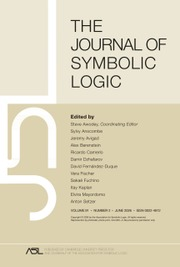Home
Hostname: page-component-55597f9d44-qcsxw Total loading time: 0.255 Render date: 2022-08-18T21:12:25.480Z Has data issue: true Feature Flags: { "shouldUseShareProductTool": true, "shouldUseHypothesis": true, "isUnsiloEnabled": true, "useRatesEcommerce": false, "useNewApi": true } hasContentIssue trueThe Journal of Symbolic Logic

# The isomorphism problem for computable Abelian p-groups of bounded length

Published online by Cambridge University Press:  12 March 2014

## Abstract

Theories of classification distinguish classes with some good structure theorem from those for which none is possible. Some classes (dense linear orders, for instance) are non-classifiable in general, but are classifiable when we consider only countable members. This paper explores such a notion for classes of computable structures by working out a sequence of examples.

We follow recent work by Goncharov and Knight in using the degree of the isomorphism problem for a class to distinguish classifiable classes from non-classifiable. In this paper, we calculate the degree of the isomorphism problem for Abelian p-groups of bounded Ulm length. The result is a sequence of classes whose isomorphism problems are cofinal in the hyperarithmetical hierarchy. In the process, new back-and-forth relations on such groups are calculated.

## Keywords

Type
Research Article
Information
The Journal of Symbolic Logic , March 2005 , pp. 331 - 345

## Access options

Get access to the full version of this content by using one of the access options below. (Log in options will check for institutional or personal access. Content may require purchase if you do not have access.)

## References

Ash, C. J., Labelling systems and r.e. structures, Annals of Pure and Applied Logic, vol. 47 (1990), pp. 99119.CrossRefGoogle Scholar
Ash, C. J. and Knight, J. F., Computable structures and the hyperarithmetical hierarchy, Elsevier, 2000.Google Scholar
Barker, E., Back and forth relations for reduced Abelian p-groups. Annals of Pure and Applied Logic, vol, 75 (1995), pp. 223249.CrossRefGoogle Scholar
Calvert, W., The isomorphism problem for classes of computable fields. Archive for Mathematical Logic, vol. 43 (2004), pp. 327336.CrossRefGoogle Scholar
Friedman, H., Simpson, S., and Smith, R., Countable algebra and set existence axioms. Annals of Pure and Applied Logic, vol. 25 (1983). pp. 141181.CrossRefGoogle Scholar
Friedman, H. and Stanley, L., A Borel reducibility theory for classes of countable structures, this Journal, vol. 54 (1989), pp. 894914.Google Scholar
Goncharov, S. S. and Knight, J. F., Computable structure and non-structure theorems, Algebra and Logic, vol. 41 (2002), pp. 351373.CrossRefGoogle Scholar
Hirschfeldt, D., Khoussainov, B., Shore, R., and Slinko, A. M., Degree spectra and computable dimensions in algebraic structures, Annals of Pure and Applied Logic, vol. 115 (2002), pp. 71113.CrossRefGoogle Scholar
Hjorth, G., Classification and orbit equivalence relations, American Mathematical Society, 1999.CrossRefGoogle Scholar
Kaplansky, I., Infinite Abelian groups, University of Michigan Press, 1969.Google Scholar
Khisamiev, N. G., Constructive Abelian p-groups, Siberian Advances in Mathematics, vol. 2 (1992), pp. 68113.Google Scholar
Lin, C., The effective content of Ulm's theorem, Aspects of effective algebra (Crossley, J. N., editor), Upside Down A Book Company, 1979, pp. 147160.Google Scholar
Lin, C., Recursively presented Abelian groups: Effective p-group theory I, this Journal, vol. 46 (1981), pp. 617624.Google Scholar
Morozov, A. S., Functional trees and automorphisms of models, Algebra and Logic, vol. 32 (1993), pp. 2838.CrossRefGoogle Scholar
Nies, A., Undecidable fragments of elementary theories, Algebra Universalis, vol. 35 (1996), pp. 833.CrossRefGoogle Scholar
Rabin, M. O. and Scott, D., The undecidability of some simple theories, preprint.Google Scholar
Richman, F., The constructive theory of countable Abelian p-groups, Pacific Journal of Mathematics, vol. 45 (1973), pp. 621624.CrossRefGoogle Scholar
Rogers, L., The structure of p-trees: Algebraic systems related to Abelian groups, Abelian group theory: 2nd New Mexico state conference. Springer-Verlag, 1976, pp. 5772.Google Scholar
Simpson, S., Subsystems of second order arithmetic. Springer-Verlag, 1999.CrossRefGoogle Scholar
20
Cited by

# Save article to Kindle

Note you can select to save to either the @free.kindle.com or @kindle.com variations. ‘@free.kindle.com’ emails are free but can only be saved to your device when it is connected to wi-fi. ‘@kindle.com’ emails can be delivered even when you are not connected to wi-fi, but note that service fees apply.

Find out more about the Kindle Personal Document Service.

The isomorphism problem for computable Abelian p-groups of bounded length
Available formats
×

# Save article to Dropbox

To save this article to your Dropbox account, please select one or more formats and confirm that you agree to abide by our usage policies. If this is the first time you used this feature, you will be asked to authorise Cambridge Core to connect with your Dropbox account. Find out more about saving content to Dropbox.

The isomorphism problem for computable Abelian p-groups of bounded length
Available formats
×

# Save article to Google Drive

To save this article to your Google Drive account, please select one or more formats and confirm that you agree to abide by our usage policies. If this is the first time you used this feature, you will be asked to authorise Cambridge Core to connect with your Google Drive account. Find out more about saving content to Google Drive.

The isomorphism problem for computable Abelian p-groups of bounded length
Available formats
×
×